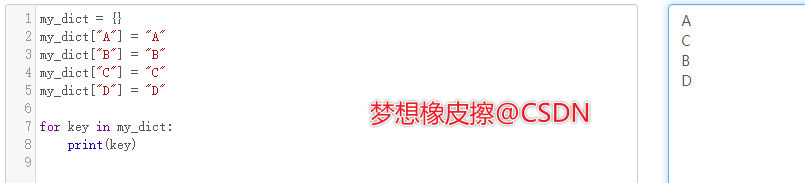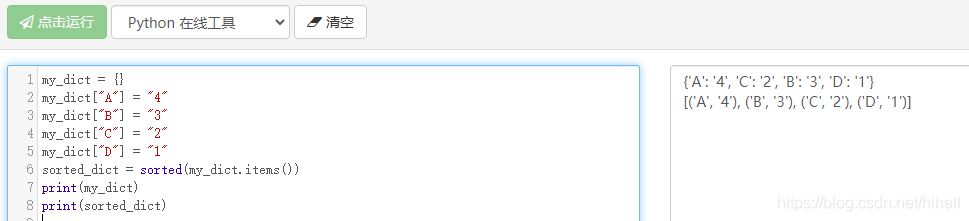# 说完列表说字典，说完字典说集合，滚雪球学 Python# 二、字典与集合那些事儿

## 2.1 字典和集合那些基础操作

### 2.1.1 先说字典

my_dict = {}my_dict["A"] = "A"my_dict["B"] = "B"my_dict["C"] = "C"my_dict["D"] = "D"for key in my_dict:    print(key)my_dict = {}my_dict["A"] = "A"my_dict["B"] = "B"my_dict["C"] = "C"my_dict["D"] = "D"print(my_dict["F"])

Traceback (most recent call last):  File ".\demo.py", line 7, in <module>    print(my_dict["F"])KeyError: 'F'

print(my_dict.get("F","None"))

### 2.1.2 再聊集合

my_set = {"A","B","C"}print(my_set)

## 2.2 字典与集合的排序

my_set = {"A","B","C"}print(my_set.pop())my_dict = {}my_dict["A"] = "4"my_dict["B"] = "3"my_dict["C"] = "2"my_dict["D"] = "1"sorted_dict = sorted(my_dict.items(),key=lambda x:x)print(sorted_dict)

[('D', '1'), ('C', '2'), ('B', '3'), ('A', '4')]

## 2.3 字典与集合的效率问题

def find_unique_weight(students):    # 声明一个统计列表    unique_list = []    # 循环所有学生数据    for id, weight in students:        # 如果体重没有在统计列表中        if weight not in unique_list:            # 新增体重数据            unique_list.append(weight)    # 计算列表长度    ret = len(unique_list)    return retstudents = [    (1, 90),    (2, 90),    (3, 60),    (4, 100)]print(find_unique_weight(students))

def find_unique_weight(students):    # 声明一个统计集合    unique_set = set()    # 循环所有学生数据    for id, weight in students:        # 集合会自动过滤重复数据        unique_set.add(weight)    # 计算集合长度    ret = len(unique_set)    return ret

import timeid = [x for x in range(1, 10000)]# 体重数据为了计算，也只能从 1 到 10000 了weight = [x for x in range(1, 10000)]students = list(zip(id, weight))start_time = time.perf_counter()# 调用列表计算函数find_unique_weight(students)end_time = time.perf_counter()print("运算时间为：{}".format(end_time - start_time))

## 2.4 这篇博客的总结### 梦想橡皮擦

6 年产品经理+教学经验，3 年互联网项目管理经验； 互联网资深爱好者； 沉迷各种技术无法自拔，导致年龄被困在 25 岁； CSDN 爬虫 100 例作者。 个人公众号“梦想橡皮擦”。

## 评论